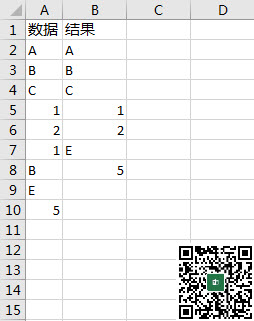﻿ 如何在excel中用函数公式提取不重复值？ - Excel - ExcelOffice【微信公众号：水星Excel】

# 如何在excel中用函数公式提取不重复值？`=IFERROR(INDEX(A:A,SMALL(IF(MATCH(\$A\$2:\$A\$10,\$A\$2:\$A\$10,0)=ROW(\$A\$2:\$A\$10)-1,ROW(\$A\$2:\$A\$10),65536),ROW(A1)))&"","")`

1.MATCH(\$A\$2:\$A\$10,\$A\$2:\$A\$10,0)把要提取不重复值的数据用Match函数求出它们各自第一次出现的位置序号，上图中是{1;2;3;4;5;4;2;8;9}

2.然后用=ROW(\$A\$2:\$A\$10)-1跟顺序的位置号匹配，相等的即为第一次出现的数据，不相等的即为重复出现的数据，上图中的结果是{TRUE;TRUE;TRUE;TRUE;TRUE;FALSE;FALSE;TRUE;TRUE}

3.用IF(MATCH(\$A\$2:\$A\$10,\$A\$2:\$A\$10,0)=ROW(\$A\$2:\$A\$10)-1,ROW(\$A\$2:\$A\$10),65536)把第一次出现的数据列出它在A列对应的行号，不是第一次出现的数据，统一把行号设定为A列的65536行（因为那行一般没有数据）

4.然后用INDEX+SMALL函数按照从小到大的顺序返回相应的内容，对于不是第一此出现的数据，返回65536行的内容，即为空数据。

5.最后用IFERROR对于超出范围的内容导致的错误留空处理。

oRng：=OFFSET(Sheet1!\$A\$1,1,,9)

`=IFERROR(INDEX(A:A,SMALL(IF(MATCH(oRng,oRng,0)=ROW(oRng)-MIN(ROW(oRng))+1,ROW(oRng),65536),ROW(A1)))&"","")`